User:Jaycall/T3 Coordinates/Special Case

Coordinate Transformations

If the special case q2 = 2 is considered, it is possible to invert the coordinate transformations in closed form. The coordinate transformations and their inversions become

 λ1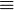$\equiv$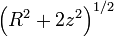$\left( R^2+2z^2 \right)^{1/2}$ and λ2$\equiv$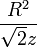$\frac{R^2}{\sqrt{2}z}$ R2$\equiv$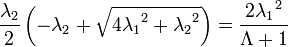$\frac{\lambda_2}{2} \left( - \lambda_2 + \sqrt{4{\lambda_1}^2 + {\lambda_2}^2} \right) = \frac{2{\lambda_1}^2}{\Lambda+1}$ and z$\equiv$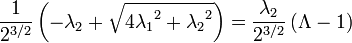$\frac{1}{2^{3/2}} \left( -\lambda_2 + \sqrt{4 {\lambda_1}^2+{\lambda_2}^2} \right) = \frac{\lambda_2}{2^{3/2}} \left( \Lambda -1 \right)$

where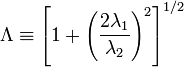$\Lambda \equiv \left[ 1 + \left( \frac{2 \lambda_1}{\lambda_2} \right)^2 \right]^{1/2}$ .

From this definition of Λ, we can compute both its partials with respect to the T3 coordinates, and its total time derivative.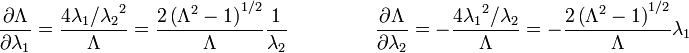$\frac{\partial \Lambda}{\partial \lambda_1} = \frac{4 \lambda_1 / {\lambda_2}^2}{\Lambda} = \frac{2 \left( \Lambda^2 - 1 \right)^{1/2}}{\Lambda} \frac{1}{\lambda_2} \ \ \ \ \ \ \ \ \ \ \ \ \ \ \ \ \ \ \ \ \frac{\partial \Lambda}{\partial \lambda_2} = - \frac{4 {\lambda_1}^2 / \lambda_2}{\Lambda} = - \frac{2 \left( \Lambda^2 - 1 \right)^{1/2}}{\Lambda} \lambda_1$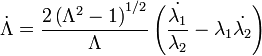$\dot{\Lambda} = \frac{2 \left( \Lambda^2 - 1 \right)^{1/2}}{\Lambda} \left( \frac{\dot{\lambda_1}}{\lambda_2} - \lambda_1 \dot{\lambda_2} \right)$

Partials of the Coordinates

Partial derivatives of each of the T3 coordinates taken with respect to each of the cylindrical coordinates are: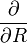$\frac{\partial}{\partial R}$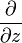$\frac{\partial}{\partial z}$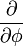$\frac{\partial}{\partial \phi}$ λ1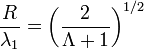$\frac{R}{\lambda_1} = \left( \frac{2}{\Lambda + 1} \right)^{1/2}$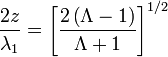$\frac{2z}{\lambda_1} = \left[ \frac{2 \left( \Lambda - 1 \right) }{\Lambda + 1} \right]^{1/2}$ 0 λ2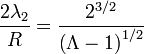$\frac{2 \lambda_2}{R} = \frac{2^{3/2}}{\left( \Lambda - 1 \right)^{1/2}}$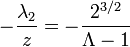$-\frac{\lambda_2}{z} = - \frac{2^{3/2}}{\Lambda - 1}$ 0 λ3 0 0 1

And partials of the cylindrical coordinates taken with respect to the T3 coordinates are: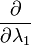$\frac{\partial}{\partial \lambda_1}$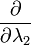$\frac{\partial}{\partial \lambda_2}$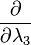$\frac{\partial}{\partial \lambda_3}$ R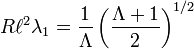$R \ell^2 \lambda_1 = \frac{1}{\Lambda} \left( \frac{\Lambda + 1}{2} \right)^{1/2}$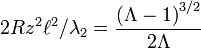$2Rz^2 \ell^2 / \lambda_2 = \frac{\left( \Lambda - 1 \right)^{3/2}}{2 \Lambda}$ 0 z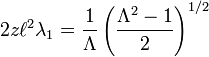$2z \ell^2 \lambda_1 = \frac{1}{\Lambda} \left( \frac{\Lambda^2 - 1}{2} \right)^{1/2}$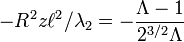$-R^2 z \ell^2 / \lambda_2 = - \frac{\Lambda - 1}{2^{3/2} \Lambda}$ 0 φ 0 0 1

where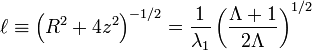$\ell \equiv \left( R^2 + 4z^2 \right)^{-1/2} = \frac{1}{\lambda_1} \left( \frac{\Lambda + 1}{2 \Lambda} \right)^{1/2}$.

Scale Factors

Furthermore, the scale factors become

 h1 =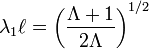$\lambda_1 \ell = \left( \frac{\Lambda+1}{2 \Lambda} \right)^{1/2}$ h2 =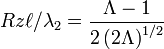$Rz \ell / \lambda_2 = \frac{\Lambda-1}{2 \left( 2 \Lambda \right)^{1/2}}$ h3 = R = λ3

Useful Relationships

In this special case, there are some additional useful relationships between various combinations of cylindrical variables and their T3 equivalents which can be written out.

 R2 + 2z2 =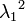${\lambda_1}^2$ R2 + 4z2 =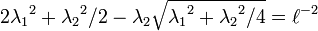$2 {\lambda_1}^2 + {\lambda_2}^2/2 - \lambda_2 \sqrt{{\lambda_1}^2+{\lambda_2}^2/4} = \ell^{-2}$ R2 + 8z2 =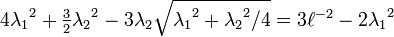$4 {\lambda_1}^2 + \tfrac{3}{2} {\lambda_2}^2 - 3 \lambda_2 \sqrt{{\lambda_1}^2+{\lambda_2}^2/4} = 3 \ell^{-2} - 2 {\lambda_1}^2$ R2 − 2z2 =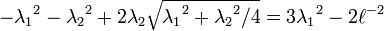$- {\lambda_1}^2 - {\lambda_2}^2 + 2 \lambda_2 \sqrt{{\lambda_1}^2+{\lambda_2}^2/4} = 3 {\lambda_1}^2 -2 \ell^{-2}$ Rz =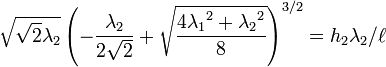$\sqrt{\sqrt{2}\lambda_2} \left( -\frac{\lambda_2}{2\sqrt{2}} + \sqrt{\frac{4{\lambda_1}^2+{\lambda_2}^2}{8}} \right)^{3/2} = h_2 \lambda_2 / \ell$

Partials of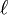$\ell$ can be taken with respect to the coordinates of either system. They are:$\frac{\partial}{\partial R}$$\frac{\partial}{\partial z}$$\frac{\partial}{\partial \phi}$$\ell$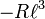$-R \ell^3$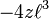$-4z \ell^3$ 0$\frac{\partial}{\partial \lambda_1}$$\frac{\partial}{\partial \lambda_2}$$\frac{\partial}{\partial \lambda_3}$$\ell$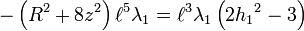$- \left( R^2 + 8z^2 \right) \ell^5 \lambda_1 = \ell^3 \lambda_1 \left( 2{h_1}^2 - 3 \right)$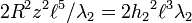$2R^2 z^2 \ell^5 / \lambda_2 = 2 {h_2}^2 \ell^3 \lambda_2$ 0

Partials of the scale factors taken with respect to the T3 coordinates are:$\frac{\partial}{\partial \lambda_1}$$\frac{\partial}{\partial \lambda_2}$$\frac{\partial}{\partial \lambda_3}$ h1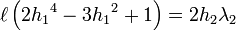$\ell \left( 2 {h_1}^4 - 3 {h_1}^2 + 1 \right) = 2 h_2 \lambda_2$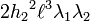$2 {h_2}^2 \ell^3 \lambda_1 \lambda_2$ 0 h2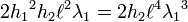$2 {h_1}^2 h_2 \ell^2 \lambda_1 = 2 h_2 \ell^4 {\lambda_1}^3$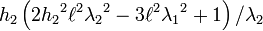$h_2 \left( 2 {h_2}^2 \ell^2 {\lambda_2}^2 - 3 \ell^2 {\lambda_1}^2 + 1 \right) / \lambda_2$ 0 h3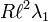$R \ell^2 \lambda_1$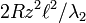$2Rz^2 \ell^2 / \lambda_2$ 0

Conserved Quantity

The conserved quantity associated with the λ2 coordinate is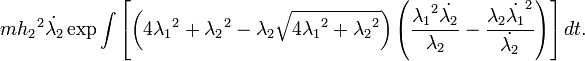$m{h_2}^2 \dot{\lambda_2} \exp \int \left[ \left( 4 {\lambda_1}^2 + {\lambda_2}^2 - \lambda_2 \sqrt{4{\lambda_1}^2 + {\lambda_2}^2} \right) \left( \frac{{\lambda_1}^2 \dot{\lambda_2}}{\lambda_2} - \frac{\lambda_2 {\dot{\lambda_1}}^2}{\dot{\lambda_2}} \right) \right] dt .$

The quantity in brackets needs to be integrated. In terms of Λ, it can be written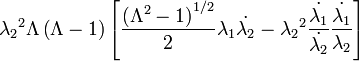${\lambda_2}^2 \Lambda \left( \Lambda - 1 \right) \left[ \frac{\left( \Lambda^2 - 1 \right)^{1/2}}{2} \lambda_1 \dot{\lambda_2} - {\lambda_2}^2 \frac{\dot{\lambda_1}}{\dot{\lambda_2}} \frac{\dot{\lambda_1}}{\lambda_2} \right]$ .

Notice that the thing in square brackets looks very closely related to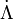$\dot{\Lambda}$. Could this be a hint? If only we could figure out what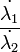$\frac{\dot{\lambda_1}}{\dot{\lambda_2}}$ is, maybe we could factor out the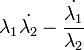$\lambda_1 \dot{\lambda_2} - \frac{\dot{\lambda_1}}{\lambda_2}$, which appears in$\dot{\Lambda}$...

If, by some miracle, it should turn out that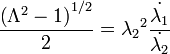$\frac{\left( \Lambda^2 - 1 \right)^{1/2}}{2} = {\lambda_2}^2 \frac{\dot{\lambda_1}}{\dot{\lambda_2}}$, factorization would be possible and our integral would read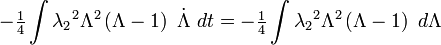$-\tfrac{1}{4} \int {\lambda_2}^2 \Lambda^2 \left( \Lambda - 1 \right) \ \dot{\Lambda} \ dt = - \tfrac{1}{4} \int {\lambda_2}^2 \Lambda^2 \left( \Lambda - 1 \right) \ d\Lambda$ .

We ought to be able to integrate this, right...? Maybe we could handle the pesky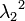${\lambda_2}^2$ with integration by parts...